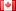# Revision history [back]

### What are the following commands telling us? R=Integers() [R.ideal([a,b]) == R.ideal([gcd(a,b)]) for a in range(1,20) for b in range(1,20)]

What are the following commands telling us? R=Integers() [R.ideal([a,b]) == R.ideal([gcd(a,b)]) for a in range(1,20) for b in range(1,20)]2 Nonefidbc2403 ●4 ●26 ●59

### What are the following commands telling us? R=Integers() [R.ideal([a,b]) == R.ideal([gcd(a,b)]) for a in range(1,20) for b in range(1,20)]

What are the following commands telling us? us?

R=Integers()
[R.ideal([a,b]) == R.ideal([gcd(a,b)])
R.ideal([gcd(a,b)]) for a in range(1,20) for b in range(1,20)]range(1,20)]